# Recap: Functions and Pattern Matching

### case classes

ex: json json objects can be seq, num, str, bool,...

⇒ represented as abstract class and case classes.

### pattern matching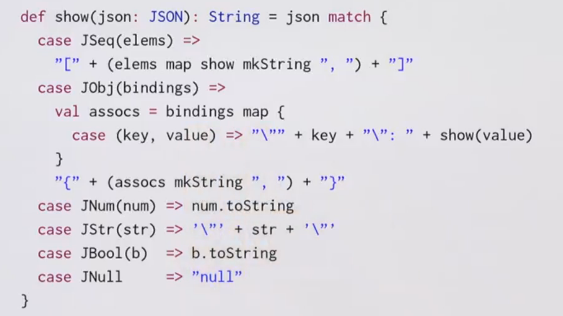→ question: what is the type of the `{case(key, value)=>"..."}` clause?

it is `(JBinding => String)` type, which is a shorthand for `Function1[JBinding, String]`.

### Function1 Trait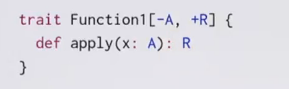### subclass a function type

function types can also be extended !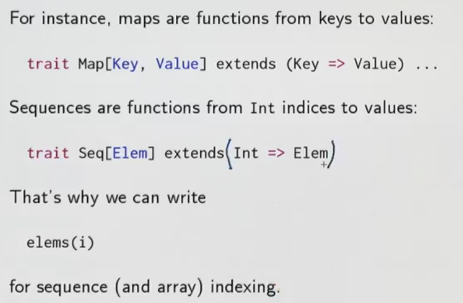element accessing can be written as function calls because `Seq`s are functions!

### Partial Match

if there is no match → throw MatchError

```val f: String=>String  = {case "ping" => "pong"}
f("ping") // no pb
f("abc") // MatchError
```

⇒ define f as partial function

```val f: PartialFunction[String,String]  = {case "ping" => "pong"}
f.isDefinedAt("ping")
f.isDefinedAt("abc")
```

`isDefinedAt` is a method for the `PartialFunction` class.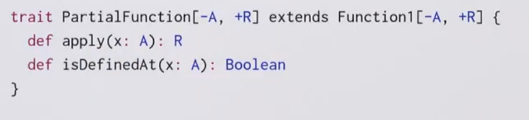The f definition is translated to: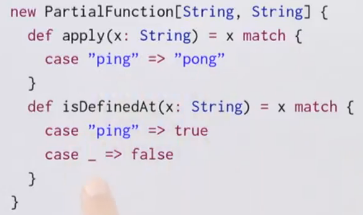But the `PartialFunction` will only apply for level 1: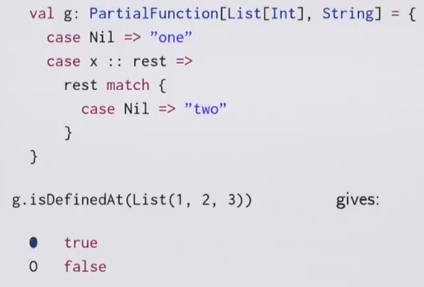# Recap: Collections

scala collections hirarchy: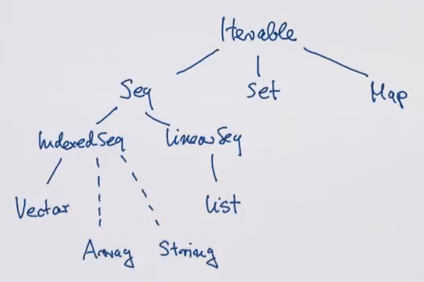collections share some general methods (core methods):

• map
• flatMap
• filter
• foldLeft/foldRight

(idealized) implementation of `map` and `flatMap` on Lists: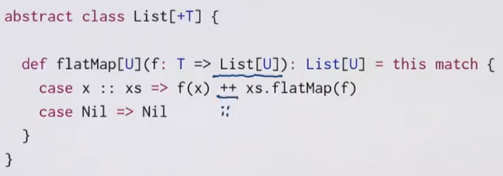### For expressions

for-expr can simplify combinations of core methods.the lhs of a generator can also be a pattern!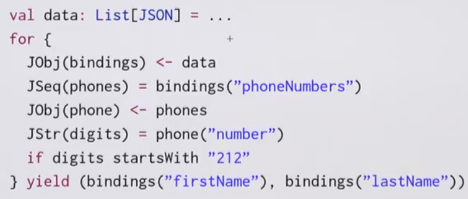pat <- expr

is translated to :

```x <- expr withFilter {case pat => true; case _ => false
} map {case pat => x}
```

# 1.1 - Queries with For

for notation is equivalent to common ops on databases(ex. sql).

ex. books in library

`case class Book(title: String, authors: List[String])`

query1: books with author name is "Bird"

```for( b<-books; a <- b.authors if a startsWith "Bird,")
yield b.title
```

query2: books with "Program" in the title:

```for( b<-books if b.title indexOf "Program" >=0)
yield b.title
```

query3: names of authors who wrote >=2 books

```for{
b1 <- books
b2 <- books
if b1!=b2
a1 <- b1.authors
a2 <- b2.authors
if a1==a2
} yield a1
```

→ pb: the authors will be doubled → b1,b2 and b2,b1 ⇒ change line 3 to `b1.title < b2.title`

→ still pb: print 3 times if authors write 3 books... ⇒ sol1. use `distinct` function sol2. decalre books as Set instead of List.

# 1.2 - Translation of For

for expressions → higer order functions

map, flatMap, filter can all be implemented with for expression: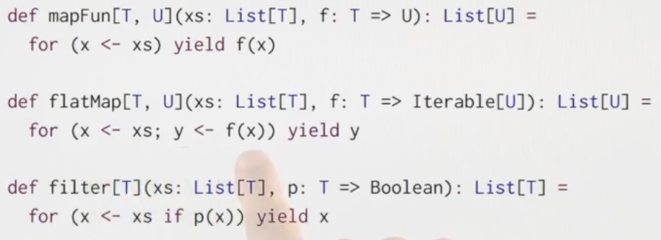In reality: scala translates for expr to map/flatMap/filter.

### implemention of for-expr: 3 rules

• rule 1: in for(..) only a simple generator

`for(x <- l1) yield e2`

is translated to: `l1.map(x => e2)`

• rule2: in for(..), followed by the generator there is a filter

`for(x <- l1 if f; s) yield e2 //s is seq of other generators and filters`

is translated to:

`for( x <- l1.withFilter(x=>f) ) yield e2`

• rule3: in for(..), starts with 2 generators → flatMap

`for( x<-l1; y<-l2; s) yield e3`

is translated to:

`l1.flatMap( x => for(y<-l2; s) yield e3 )`

example:

```for{
b <- books
a <- b.authors if a startsWith "Bird"
} yield b.title
```

translated to:

```books flatMap (
b => b.authors.filter( a => a startsWith "Bird").map(y=>y.title)

)
```

NB: for expr is not restricted to collections, it supports any types with `map/flatMap/withFilter` method. ⇒ use for expr for your own types as well.

# 1.3 - Functional Random Generators

goal: use for expr on rand generators.

for expr support any type with map/flatMap/filter ⇒ ex. rand value generator.

generate rand value of type T:

```trait Generator[+T] {
def generate: T
}
```

first implement Generator[Int], then use this to implement booleans, pairs, lists, sets, trees,......

```val integers = new Generator[Int]{
val rand = new java.util.Random
def generate = rand.nextInt()
}

val booleans = new Generator[Boolean{
def generate = integers.generate > 0
}

val pairs = new Generator[(Int, Int)]{
def generate = (integers.generate, integers.generate)
}
```

→ can we avoid the `new Generator[...]` ?

ideally we want to write booleans as pairs as:

```val booleans = for( x <- integers ) yield x>0
def pairs[T,U](t: Generator[T], u: Generator[U]) =
for{x <- t; y <- u} yield (x,y)
```

the for expr will be translated to map/flatMap/filter...

⇒ define map and flatMap on the Generator trait so that it supports for expr!

```trait Generator[+T]{
self => // syntax: `self` is an alias of `this`
def generate: T

def map[S](f: T=>S): Generator[S] =
new Generator[S]{
def generate = f(self.generate) // can't use `this` here: inf loop
// or use Generator.this.generate
}

def flatMap(f: T=>Generator[S]): Generator[S] =
new Generator[S]{
def generate = f(self.generate).generate
}
}
```

ex. the booleans expression:

`val booleans = for (x<-integers) yield x>0`

is translated to:

`val booleans = integers map (x=>x>0)`

which is then expands to:

```val booleans =
new Generator[Boolean]{
val f = (x => x>0)
def generate = f(integers.generate)

}
```

after reduction, the expression is:

```val booleans = new Generator[Boolean{
def generate = integers.generate > 0
}
```

which is the initial implementation...

### other base Generators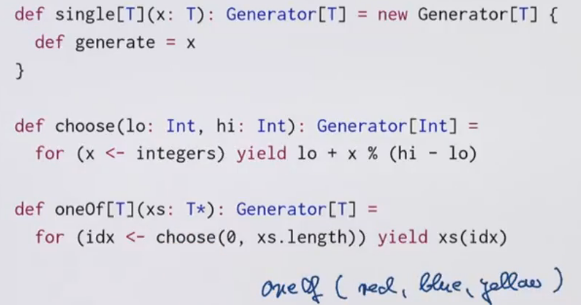(The `T*` syntax is variable parameter)

### rand List Generator

```def lists: Generator[List[Int]] = for{
isEmpty <- booleans
list <- if (isEmpty) emptyLists else nonEmptyLists
} yield list

def emptyLists = single(Nil)

def nonEmptyLists = for{
tail <- lists // recursive call to `lists`
```

### rand (binary) Tree Generator

two types of tree nodes: leaf or inner node

```def trees: Generator[Tree] = for{
isLeaf <- booleans
tree <- if(isLeaf) leafs else inners
} yield tree

def leafs: Generator[Leaf] = for{
x <- integers
} yield Leaf(x)

def inners: Generator[Inner] = for{
l <- trees
r <- trees
} yield Inner(l, r)
```

## Application: random testing

test: check postconditions (expected results)

→ generate random test inputs

a generic wrapper:

```def randTest[T](g: Generator[T], numTimes: Int=100)(testfcn: T=>Boolean): Unit = {
for( i <- 0 until numTimes){
val value:T = g.generate
assert(testfcn(value), "test failed for "+value)
}
println("passed" + numTime + "tests")
}
```

### ScalaCheck

instead of writing tests, write properties that are assumed to hold.

```forAll {
(l1:List[Int], l2:List[Int]) =>
l1.size + l2.size == (l1++l2).size
}
```

from last section: not only collections, but also any type with map and flatmap can use for expr ⇒ monads.

. Monads is a type M[T] with 2 operations: `flatMap` ("bind") and `unit`, and satisfy some laws.

```train M[T]{
def flatMap[U](f: T=>M[U]): M[U]): M[U]
}
def unit[T](x:T): M[T]
```

examples:

• `List` is a monad, `unit(x) = List(x)`
• `Set`, with `unit(x) = Set(x)`
• `Option`, with `unit(x) = Some(x)`
• `Generator`, with `unit(x) = single(x)`

`map` can be defined as a combination of `flatMap` and `unit`:

`m map f == m flatMap (x => unit( f(x)) )`

• associativity

`(m flatMap f) flatMap g == m flatMap ( x => f(x) flatMap g )`

↔ (x+y+z) = x+(y+z)

• left unit

`unit(x) flatMap f == f(x)`

• right unit

`m flatMap unit == m`

## the Try type

We define a Try class, which is similar to Option class.

```abstraxt class Try[+T]
case class Success[T](x:T) extends Try[T]
case class Failure(ex: Exception) extends Try[Nothing]
```

we can write `Try(expr)` to give a computation a try, by implementing the `apply` method of object Try:

```object Try{
def apply[T](expr: =>T): Try[T]= // expr is passed BY NAME, otherwise will cause exception in eval
try Success(expr) // java syntax of try-catch
catch{ case NonFatal(ex) => Failure(ex) }
}
}
```

if Try is a Monad ⇒ can be written in for expr:⇒ define map and flatMap on `Try` type.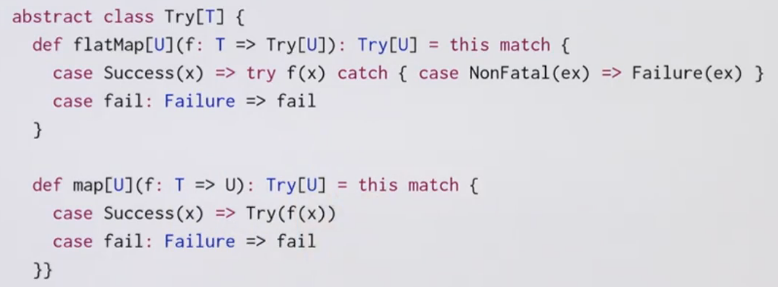question: is Try a monad with `unit(x)=Try(x)`? ⇒ no, left-unit fails: `Try(expr) flatMap f != f(expr)` (lhs never nonfatal exception, but rhs will raise)

Try is not a monad, but it can still use for expr...

### Conclusion

for exprs are useful not only for collections: `Generator`, `Option`, `Try`